## One-sided Bridged Polytans of Order 1..3

In my section about bridged polytans some constructions with pieces of order 1..3 are shown. Later I printed the 195 pieces with a total area of 284 squares using left filament of different colors. single tan: yellow, two tans: violet, three tan: green and red.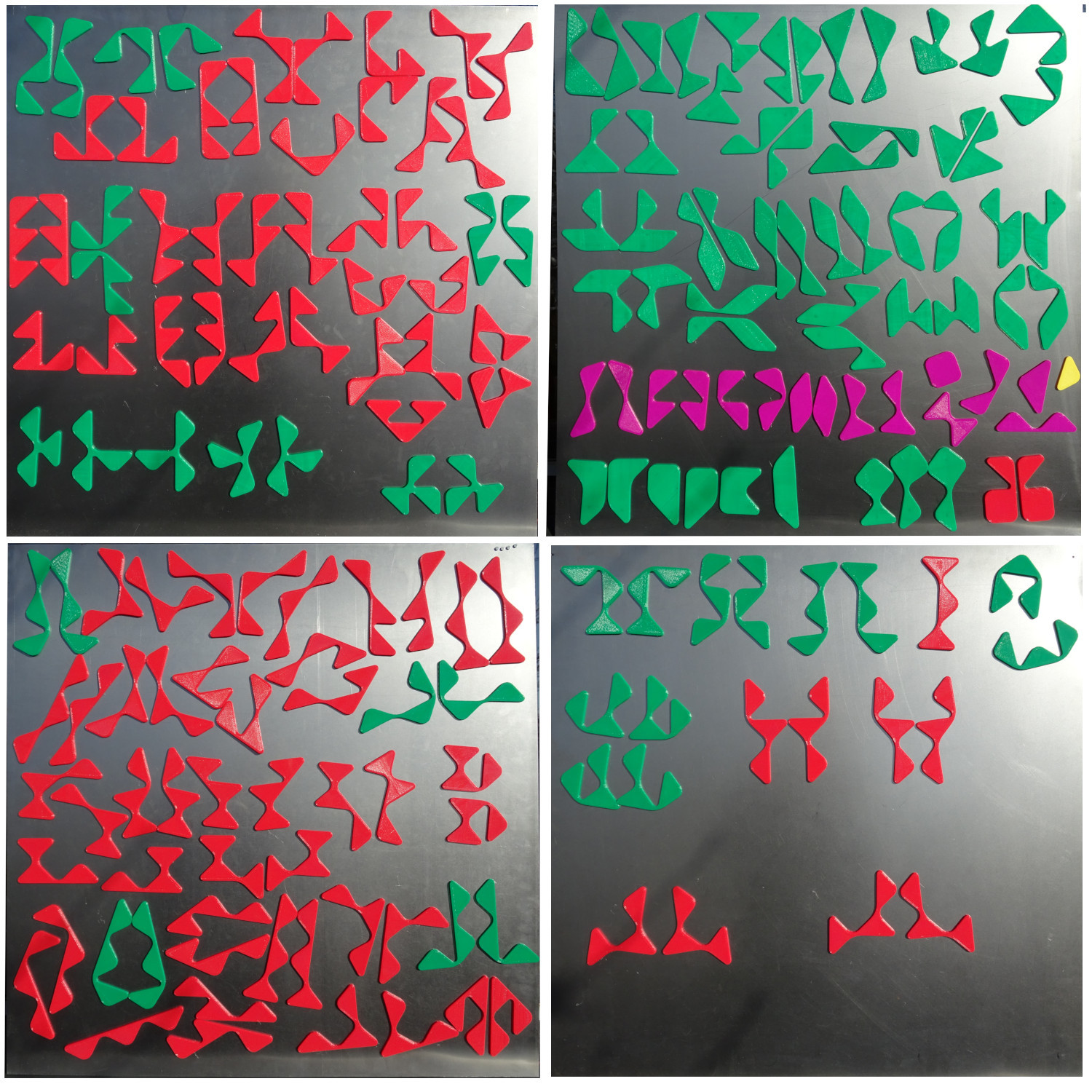Then I wanted to construct some figures, which can be scaled down by a factor of sqrt(2) to get two similar ones. A square of size 12*sqrt(2) with a hole shaped like a ditan can be made. I chose the square with the hourglass hole as an example.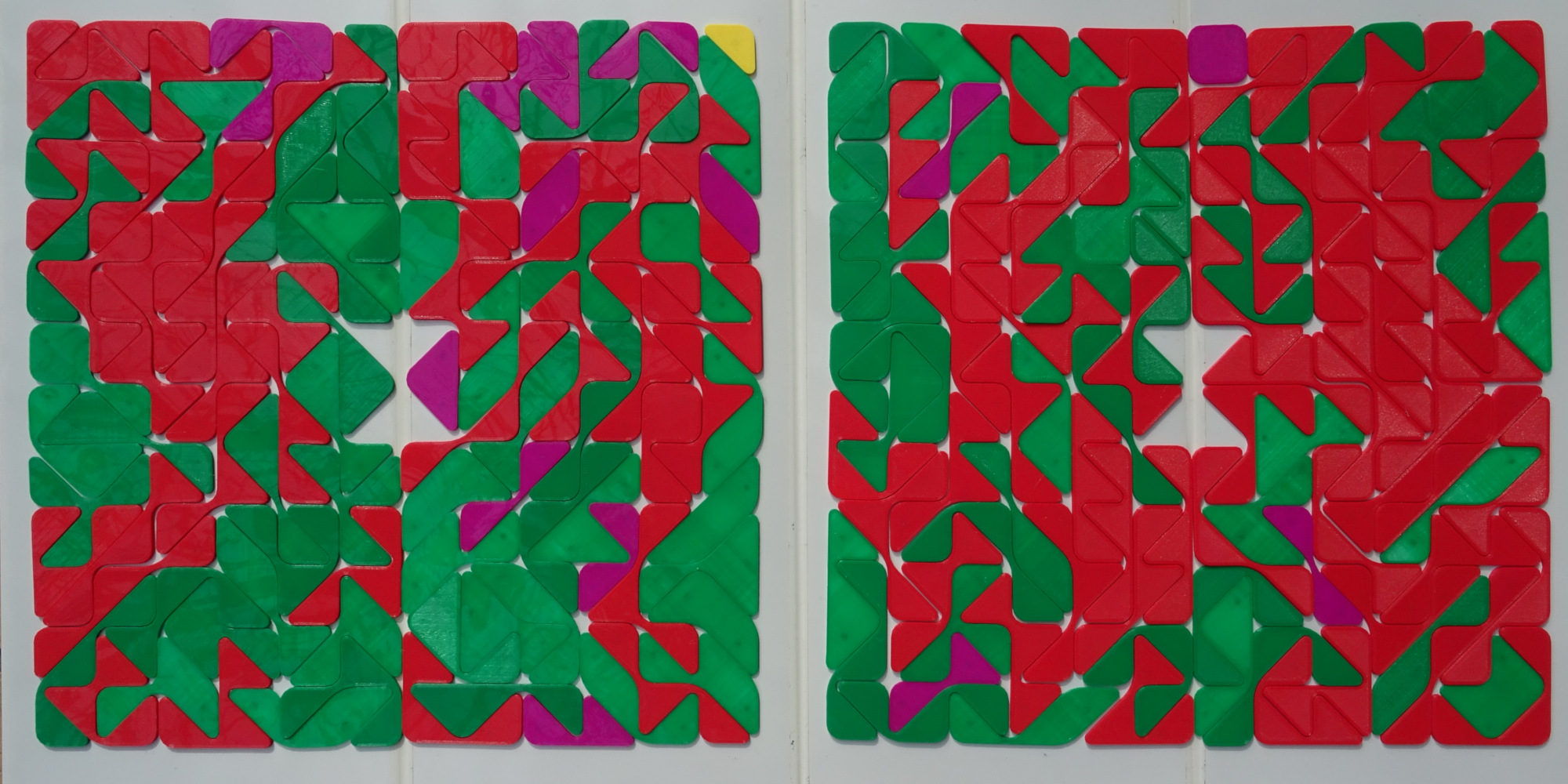A figure with square symmetry is a square ring of size 13*sqrt(2) with a square hole and 4 triangles removed at each corner.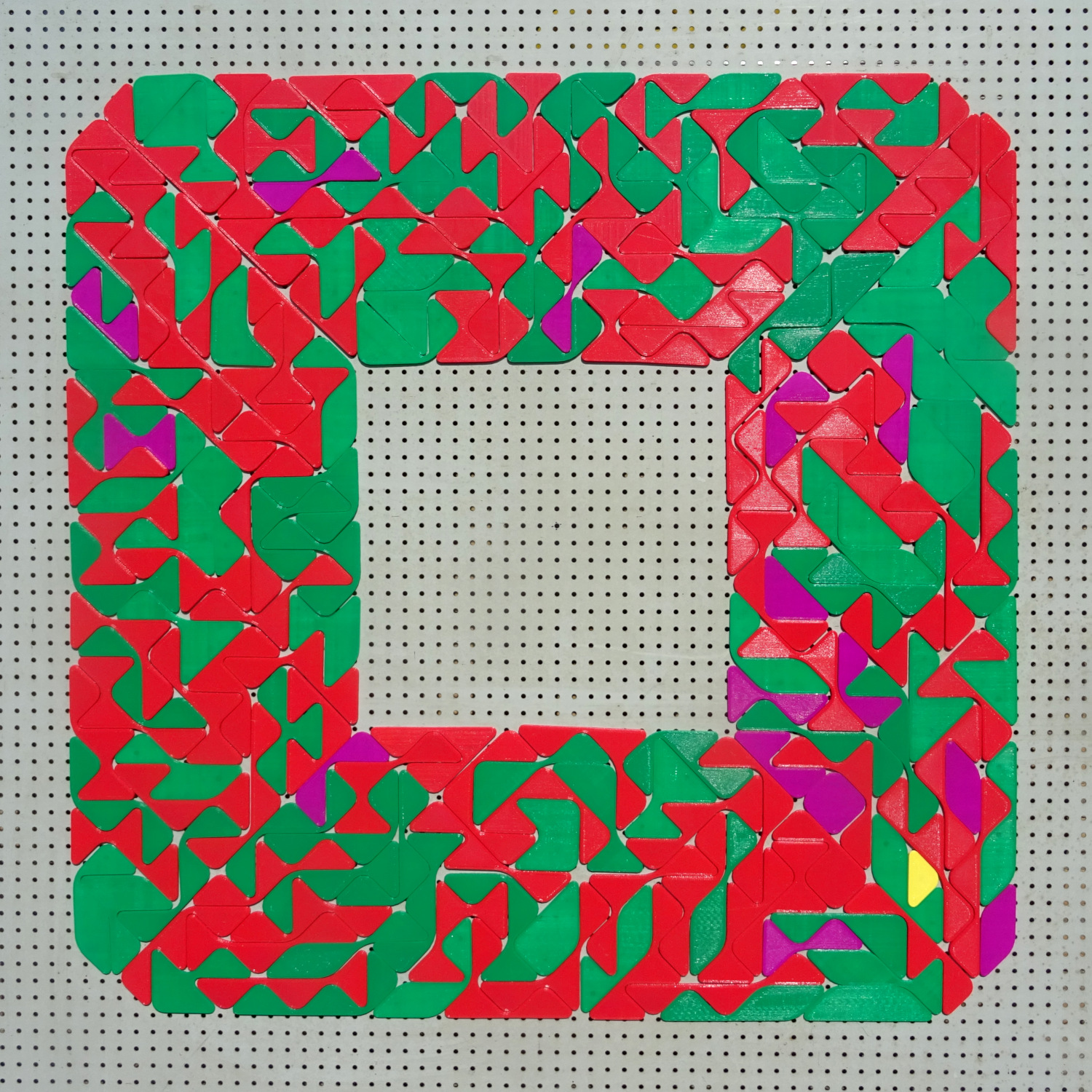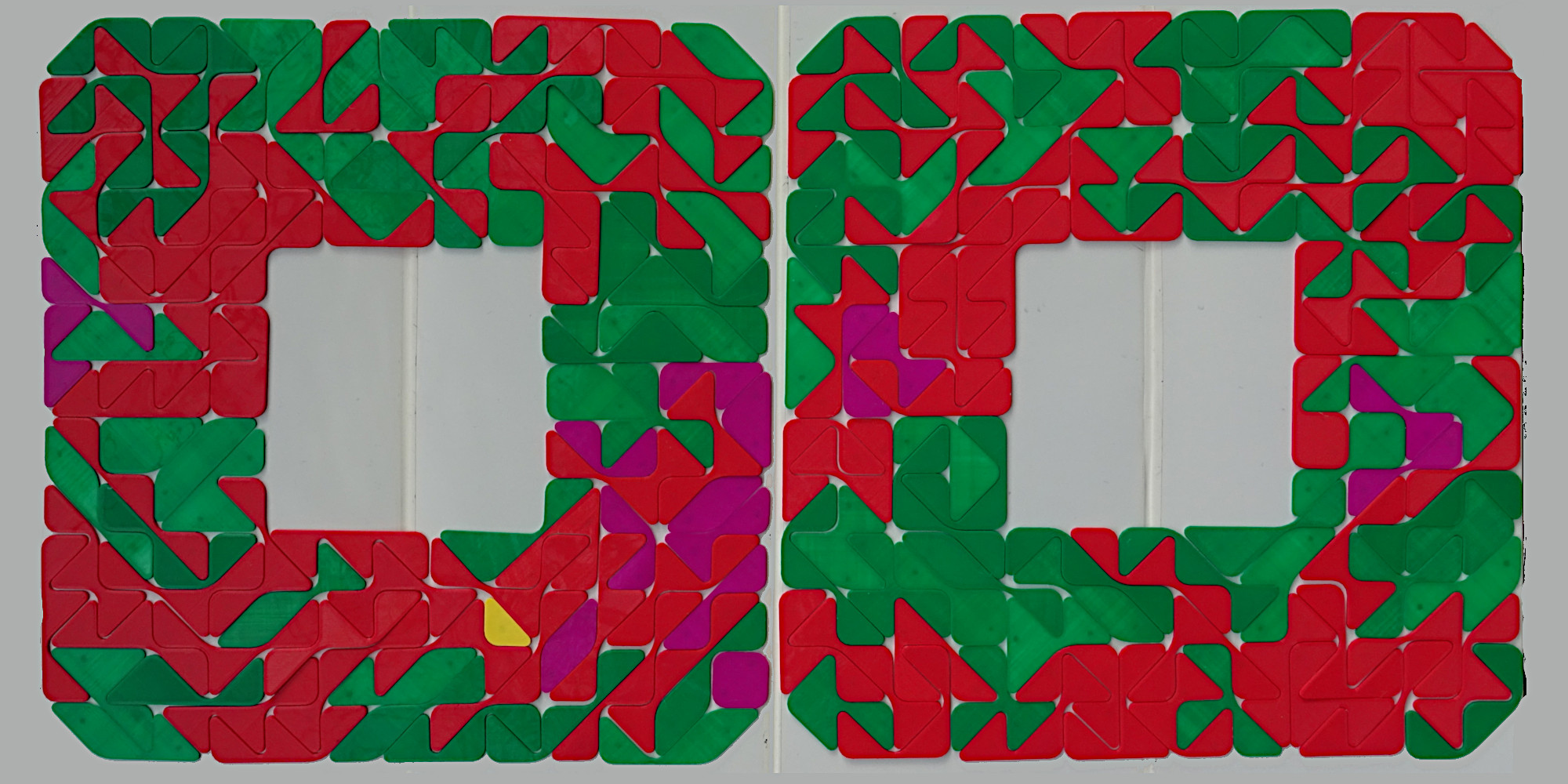The cross has also square symmetry but no holes.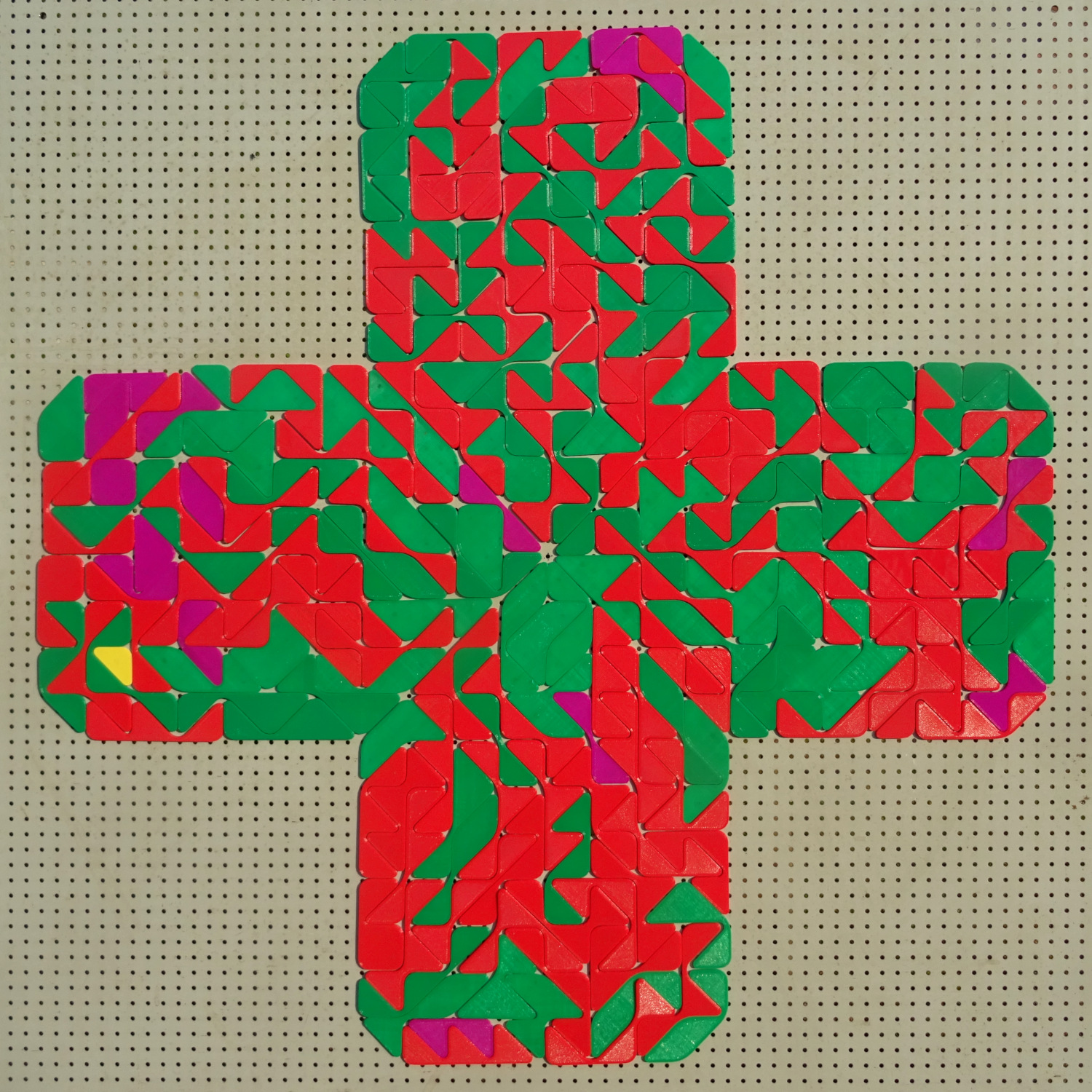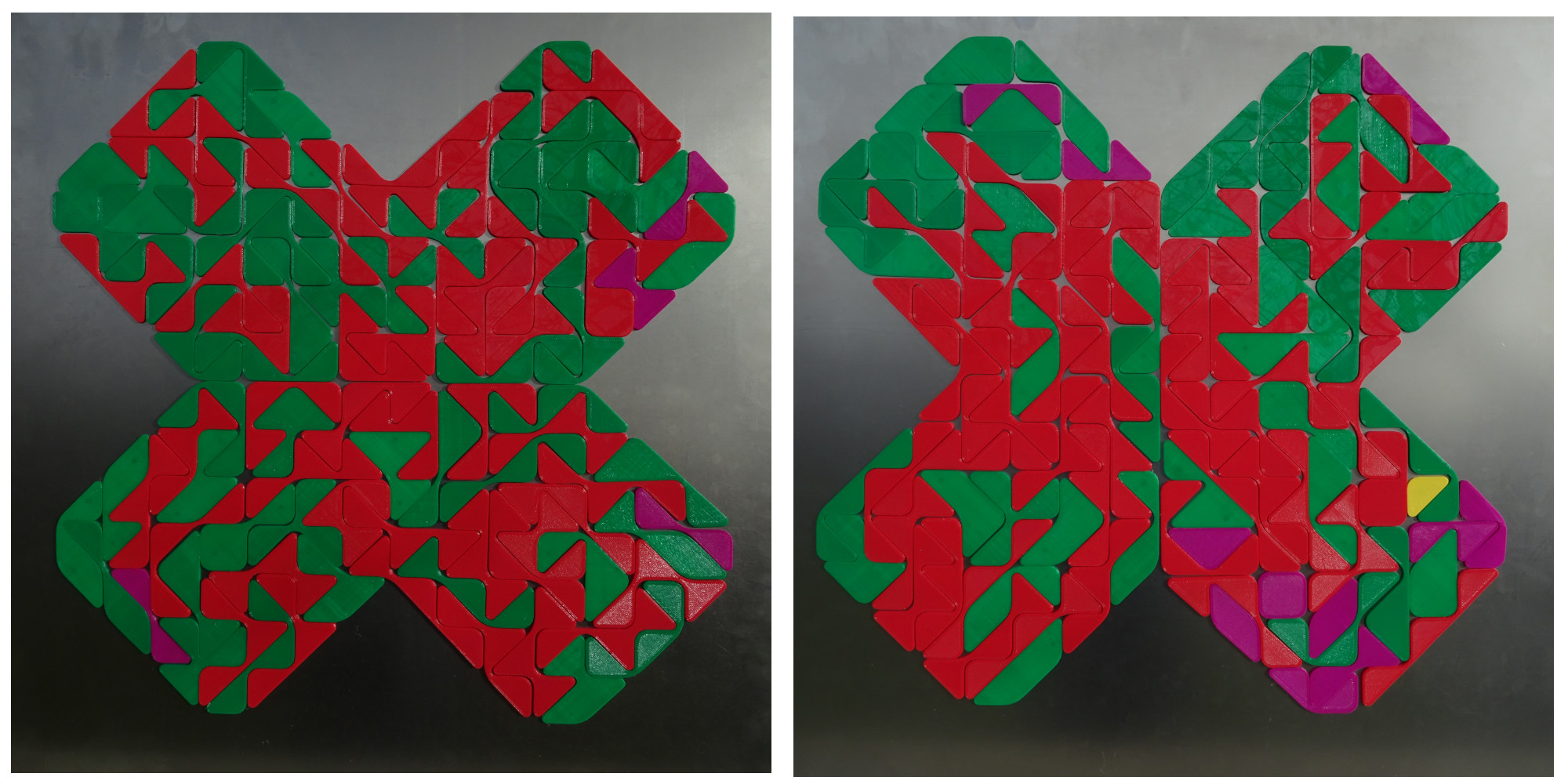A star shaped like a hexatan with a square hole is symmetric under 90 degree rotation.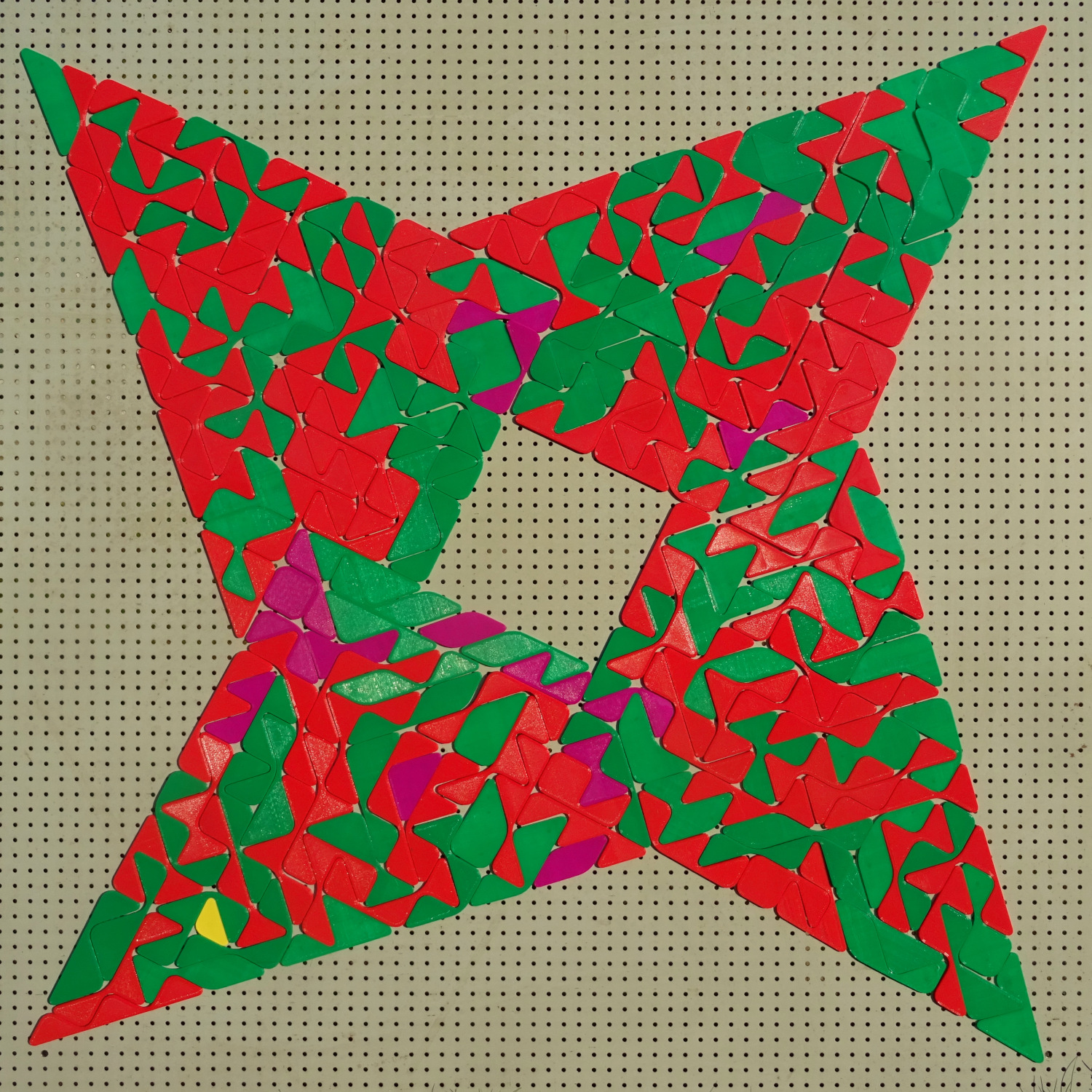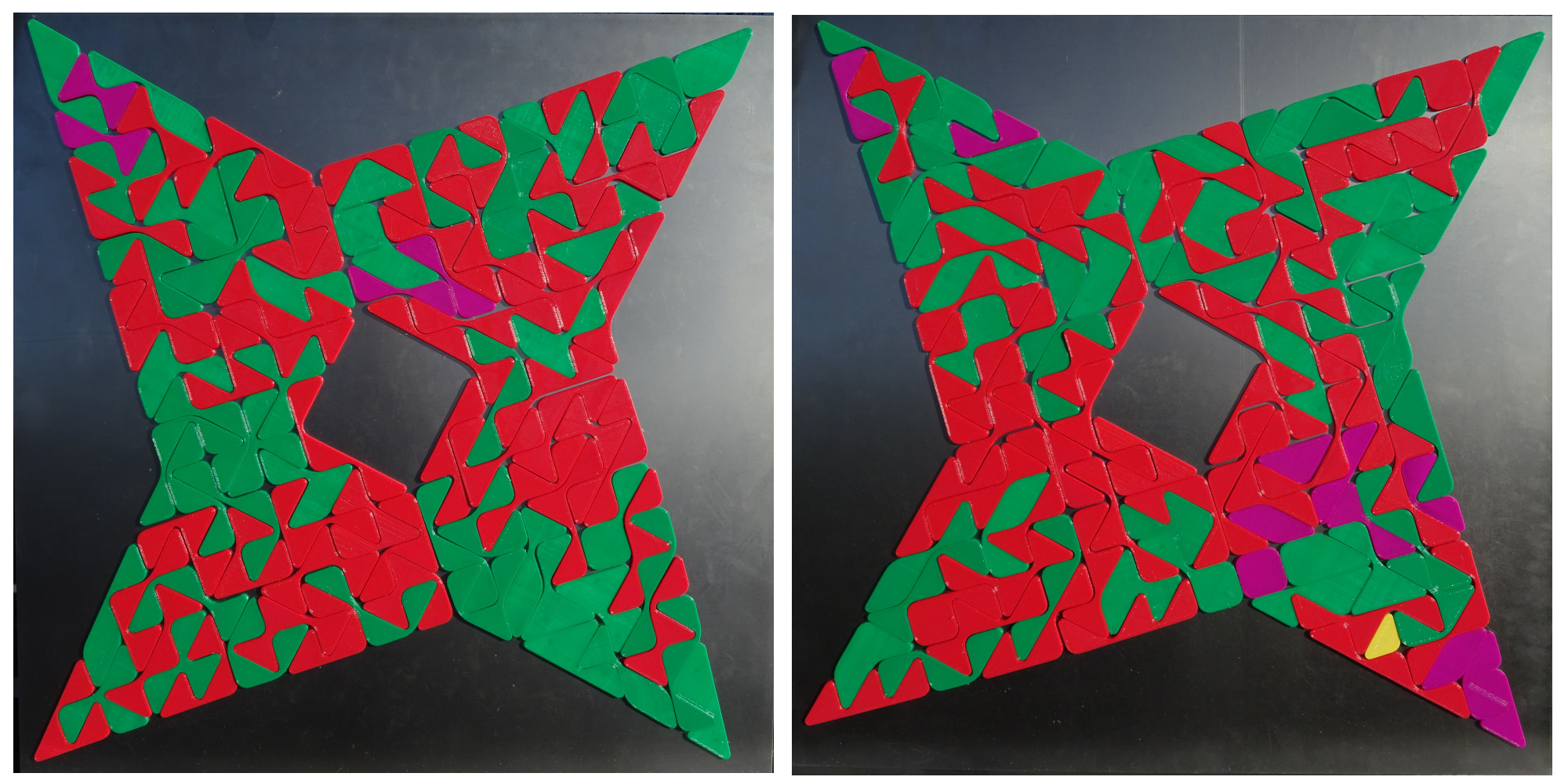For the star with eight spokes two scaled down copies aren't possible, because at the spokes a single tan is missing.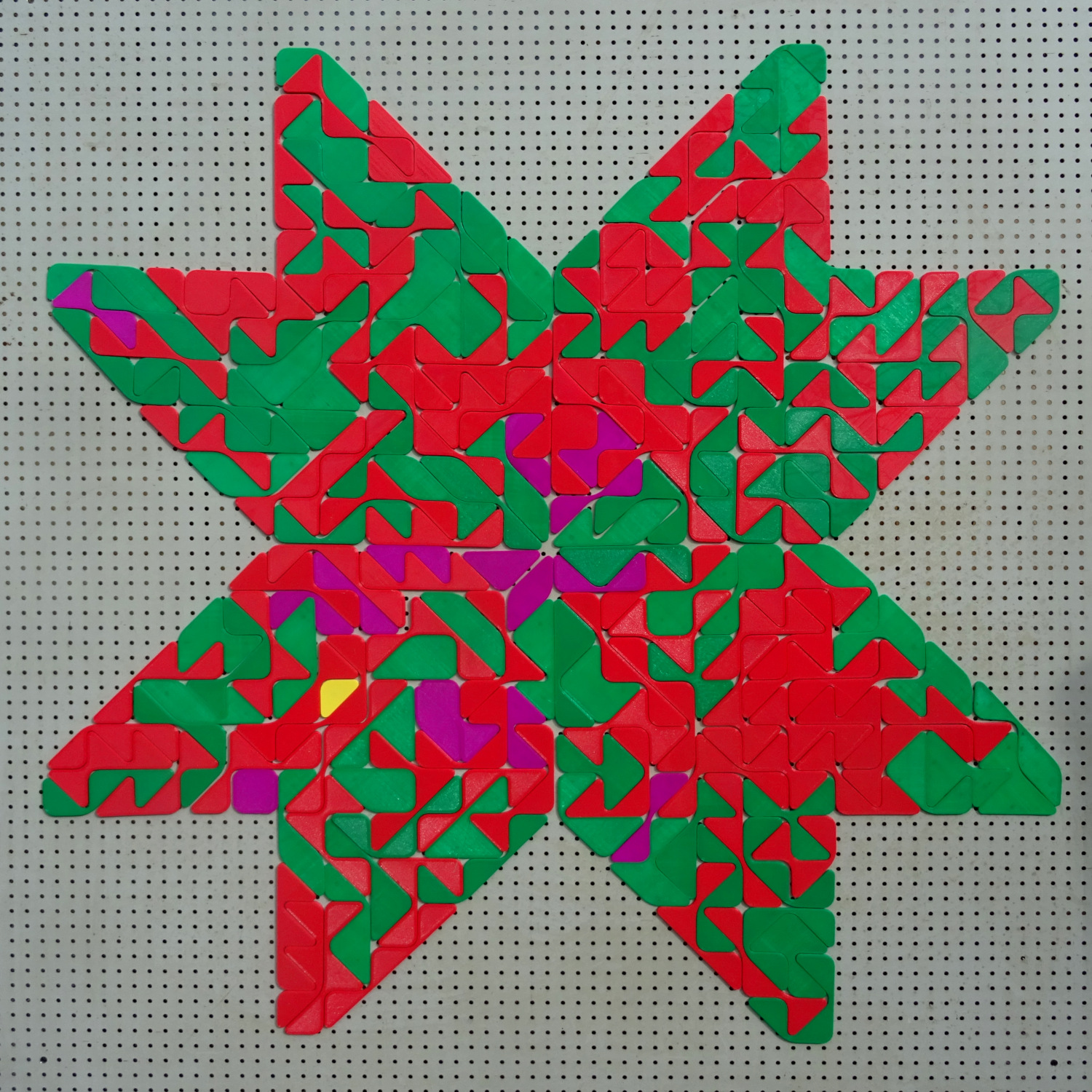Index